# RD Sharma Solutions for Class 8 Maths Chapter 19 Visualising Shapes Exercise 19.2

Exercise 19.2 of Chapter 19, mainly deals with visualisation of 3-D shapes through nets. The problems are solved in a simple and precise manner by a set of expert faculty team at BYJU’S. The solutions are designed stepwise as per the marks allotted for each step in the exam pattern, so following the steps is a must. Students can achieve their goals only by practising regularly. RD Sharma Class 8 Solutions which is a very useful study material helps students in their exam preparation. The pdf can be downloaded from the available links mentioned below.

## Download the Pdf of RD Sharma Solutions for Class 8 Maths Exercise 19.2 Chapter 19 Visualising Shapes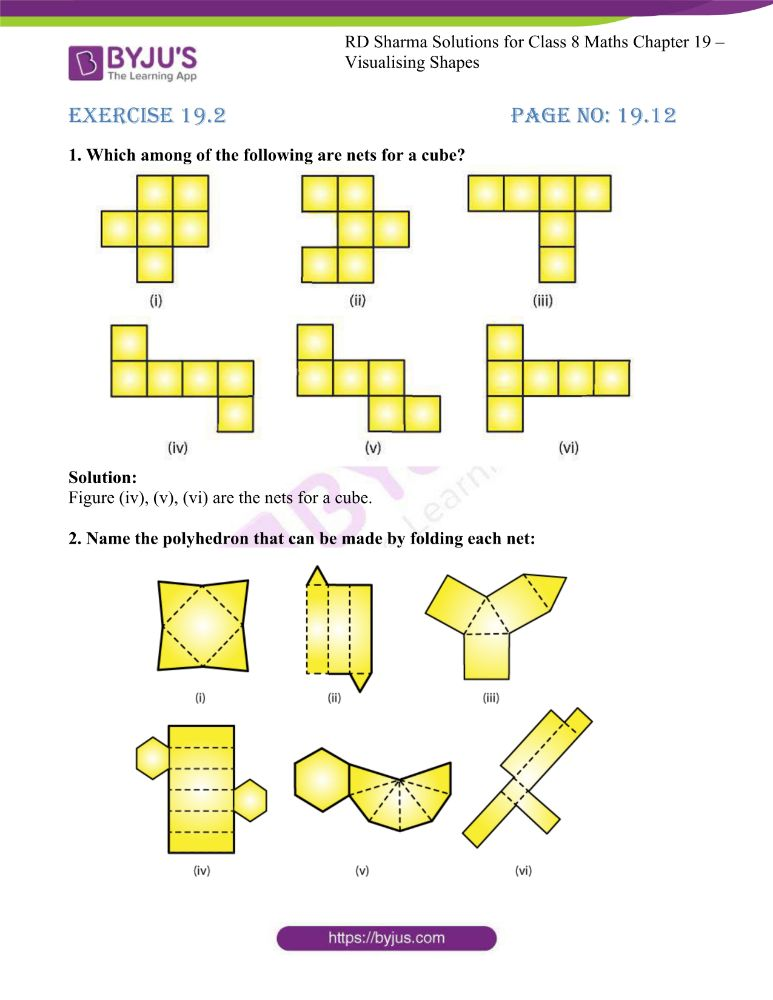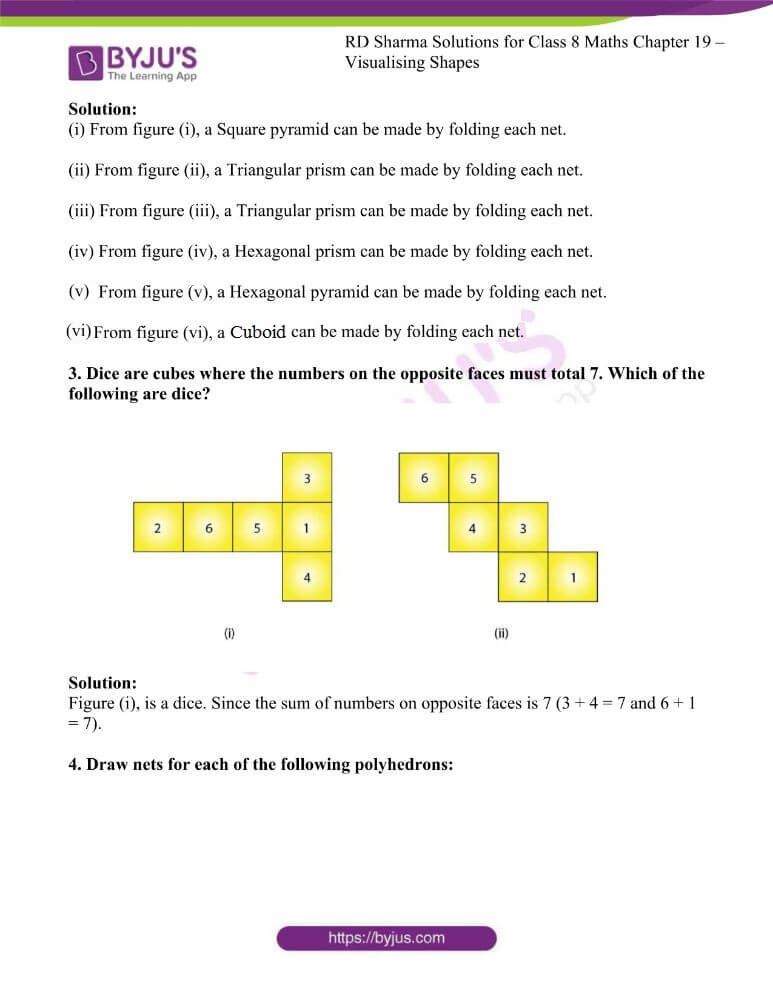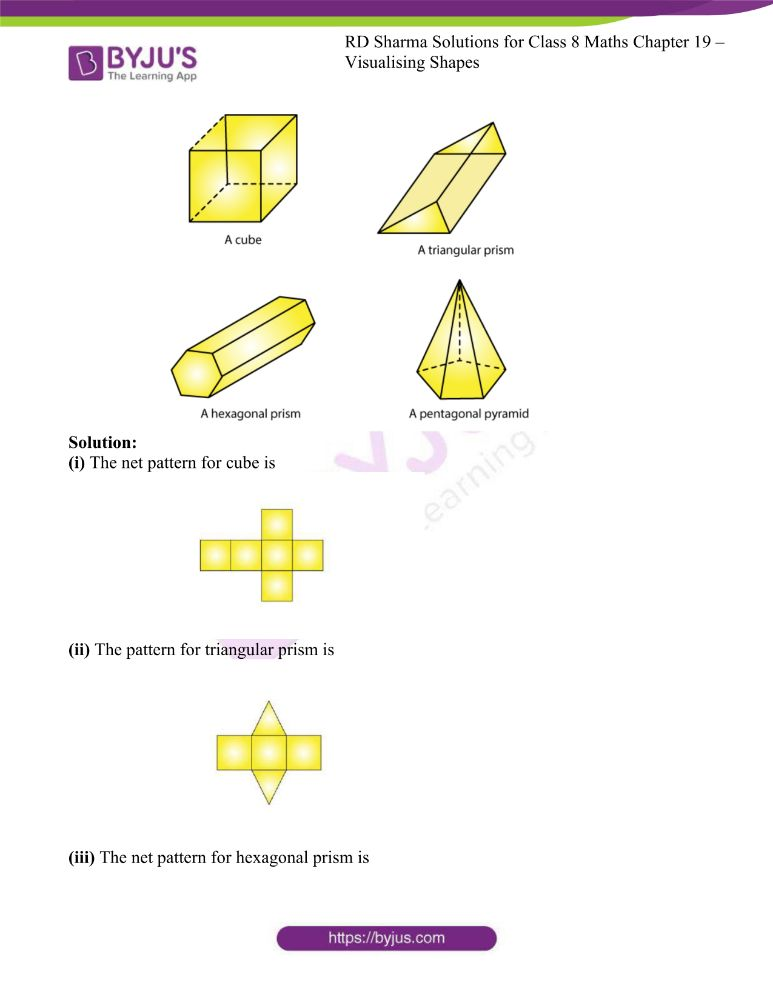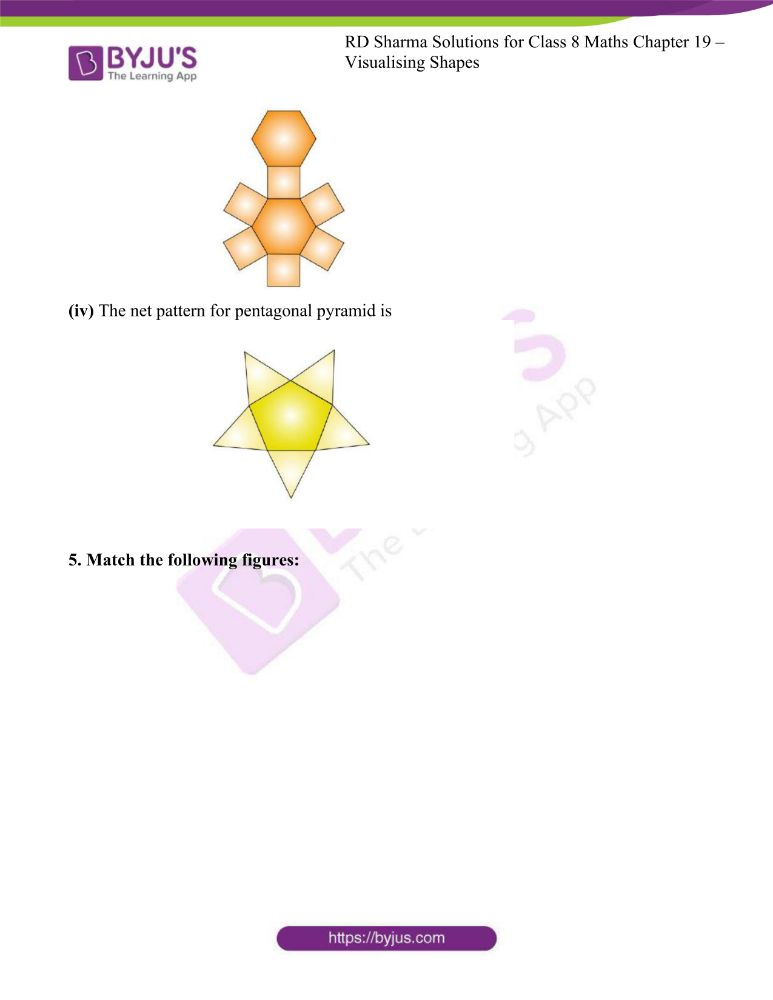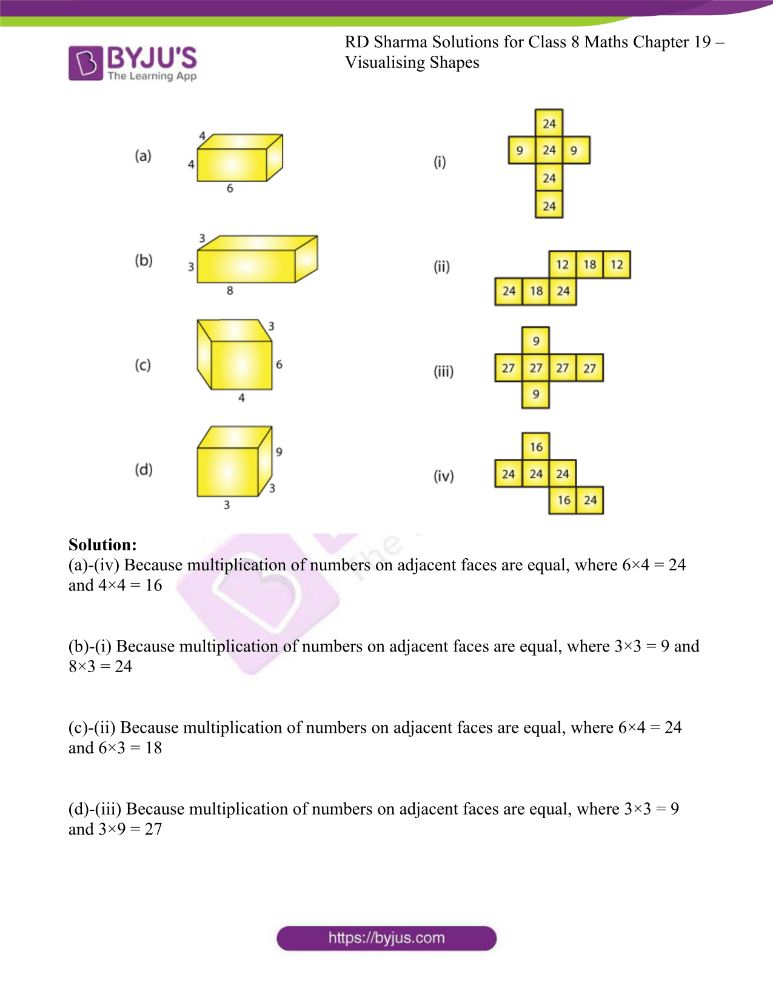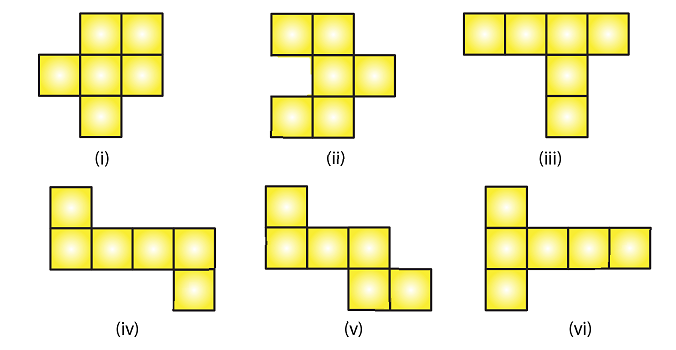Solution:

Figure (iv), (v), (vi) are the nets for a cube.

2. Name the polyhedron that can be made by folding each net: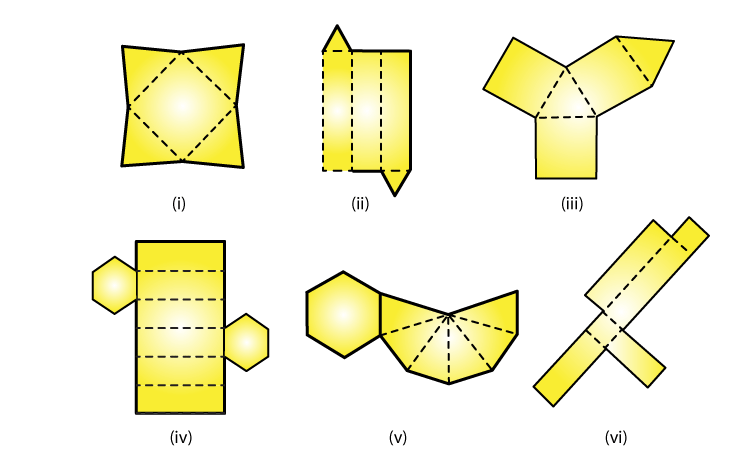Solution:

(i) From figure (i), a Square pyramid can be made by folding each net.

(ii) From figure (ii), a Triangular prism can be made by folding each net.

(iii) From figure (iii), a Triangular prism can be made by folding each net.

(iv) From figure (iv), a Hexagonal prism can be made by folding each net.

(v) From figure (v), a Hexagonal pyramid can be made by folding each net.

(vi) From figure (vi), a Cuboid can be made by folding each net.

3. Dice are cubes where the numbers on the opposite faces must total 7. Which of the following are dice?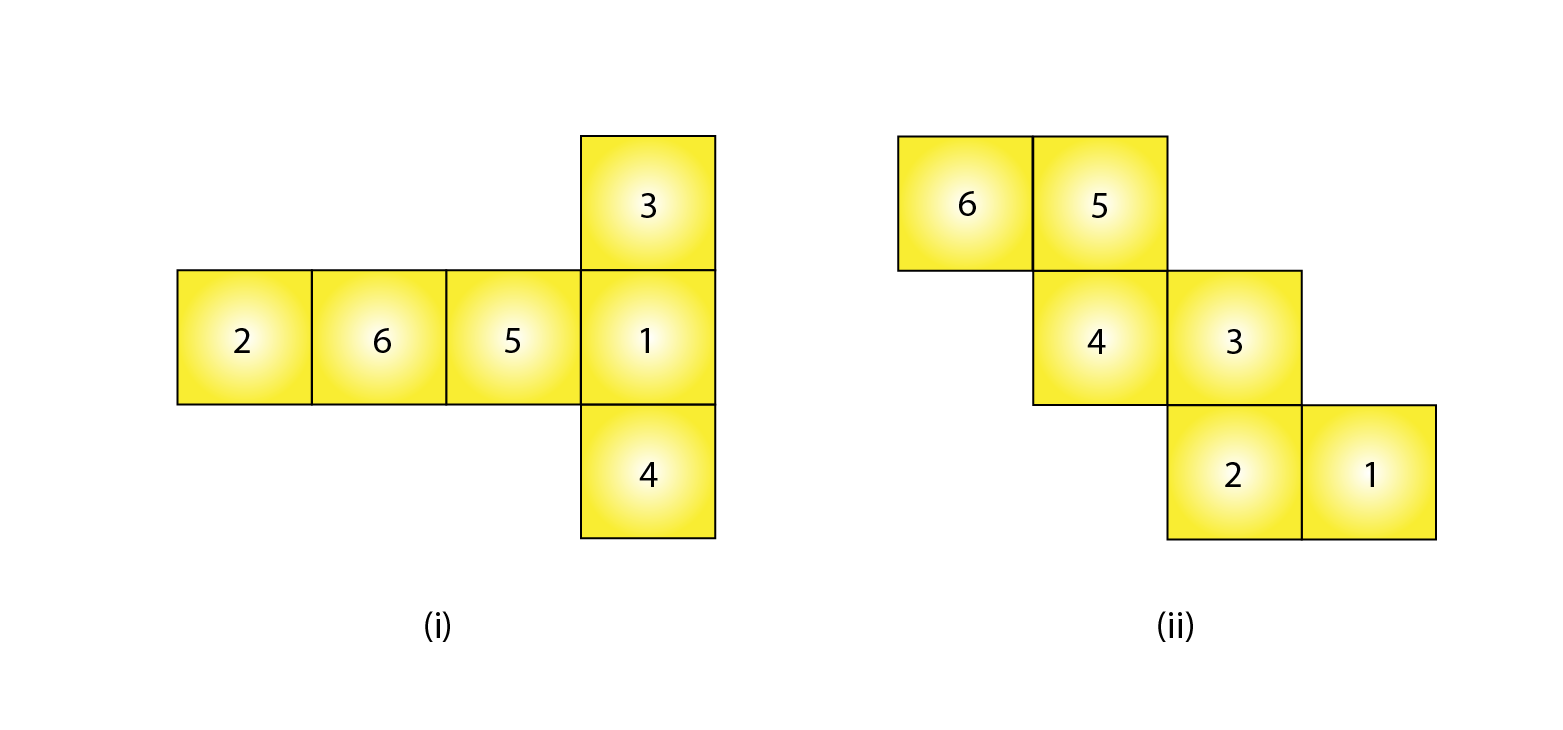Solution:

Figure (i), is a dice. Since the sum of numbers on opposite faces is 7 (3 + 4 = 7 and 6 + 1 = 7).

4. Draw nets for each of the following polyhedrons: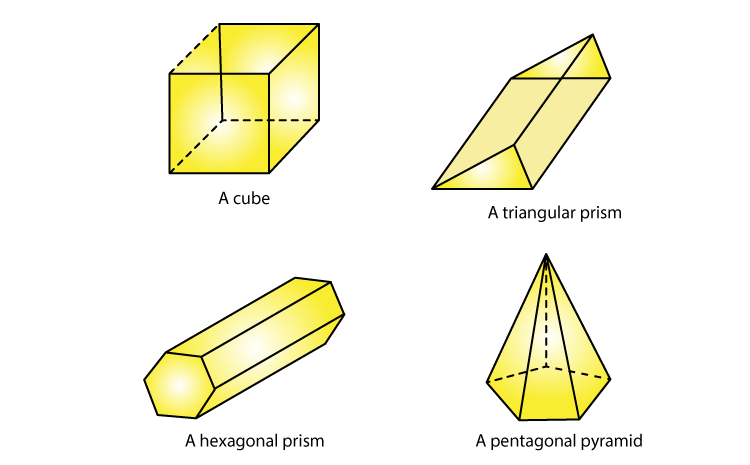Solution:

(i) The net pattern for cube is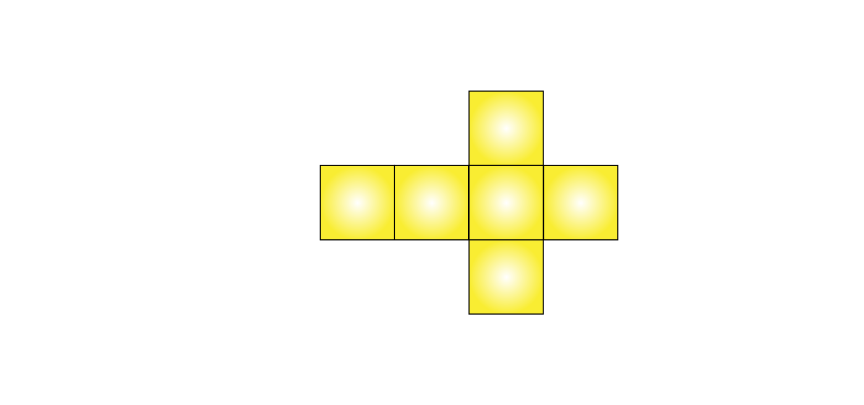(ii) The pattern for triangular prism is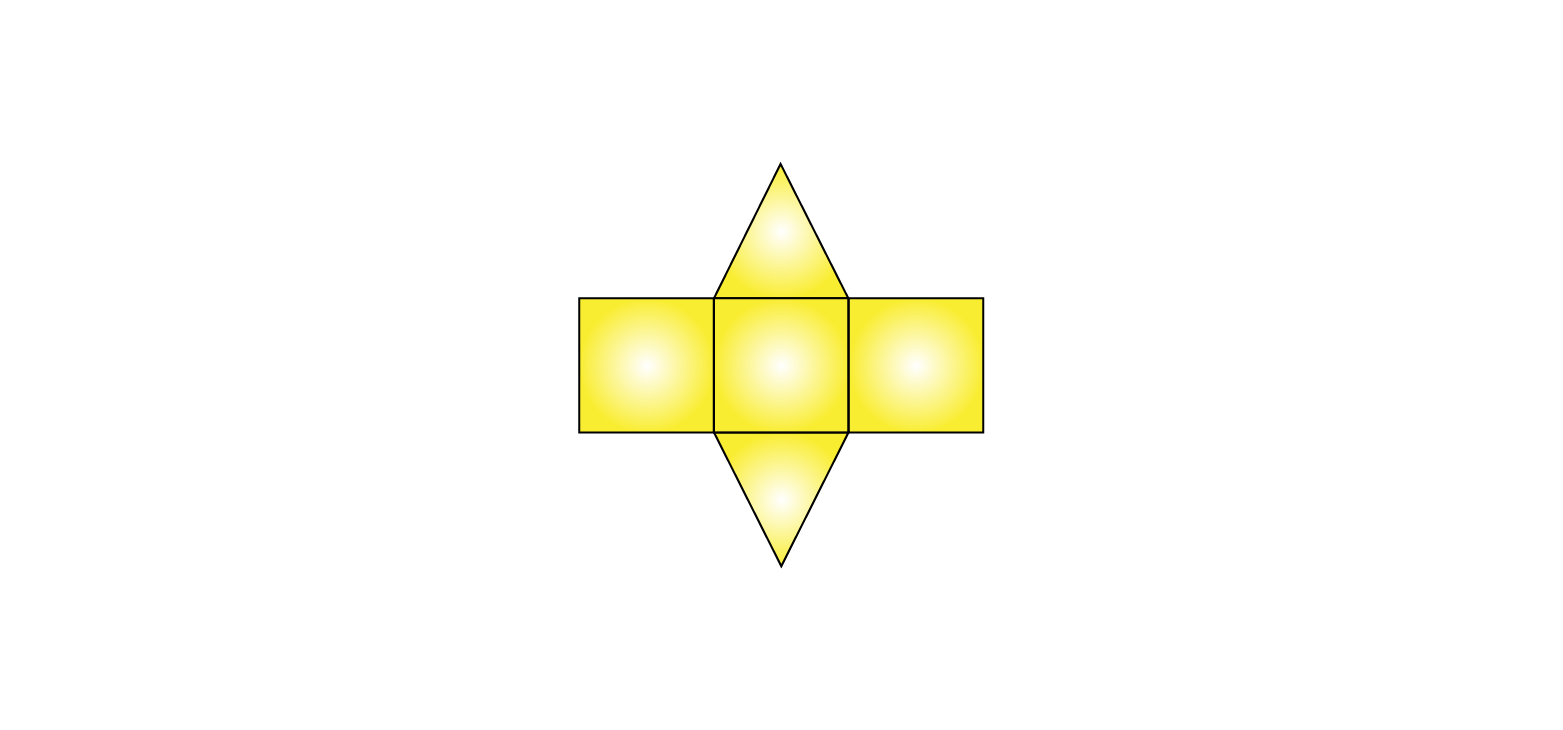(iii) The net pattern for hexagonal prism is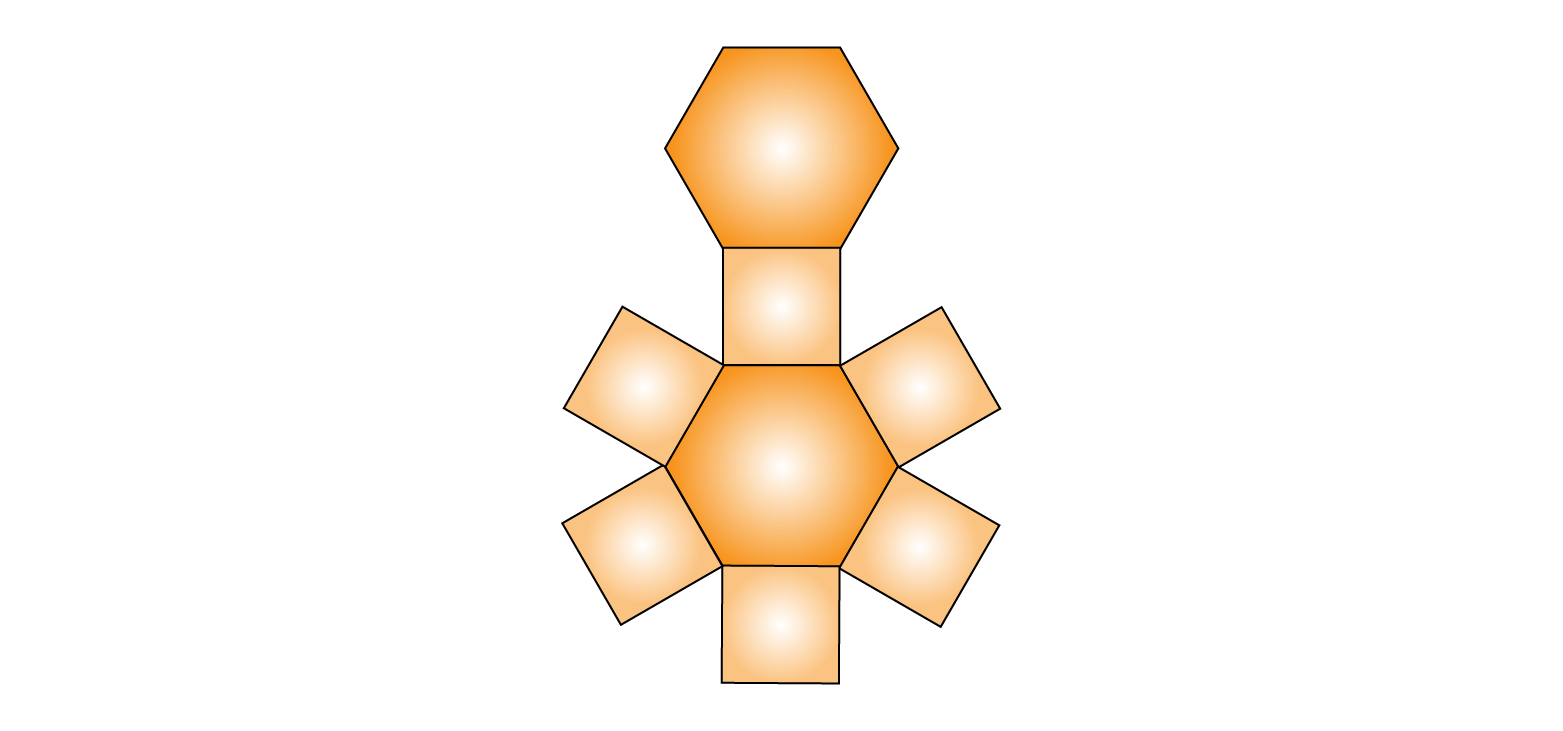(iv) The net pattern for pentagonal pyramid is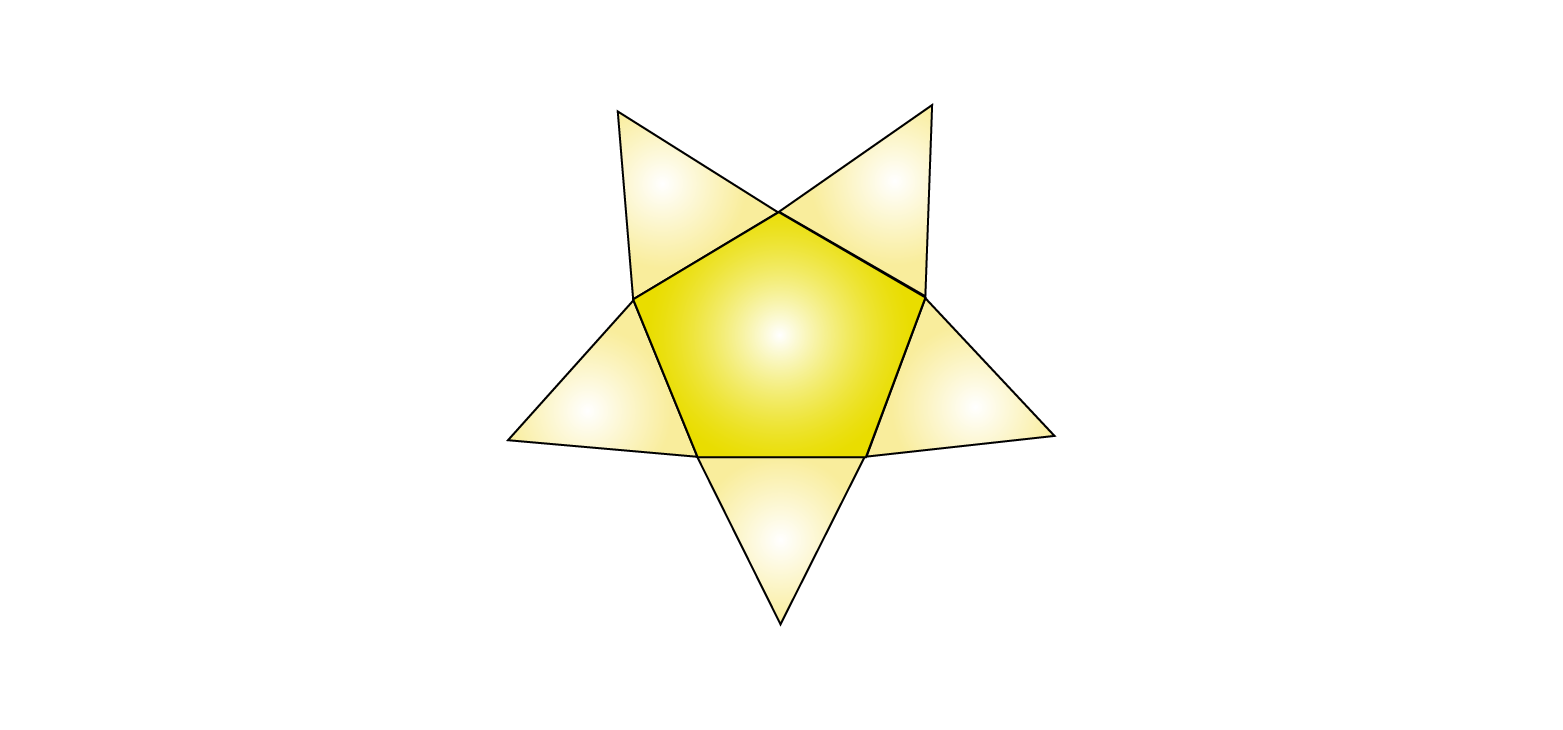5. Match the following figures: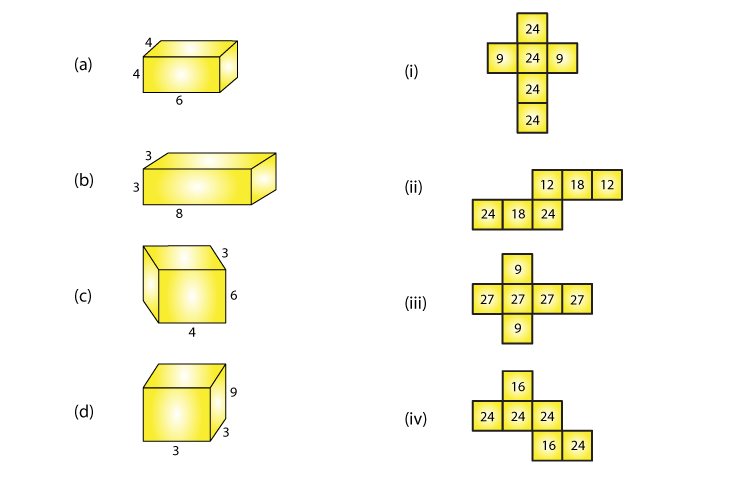Solution:

(a)-(iv) Because multiplication of numbers on adjacent faces are equal, where 6×4 = 24 and 4×4 = 16

(b)-(i) Because multiplication of numbers on adjacent faces are equal, where 3×3 = 9 and 8×3 = 24

(c)-(ii) Because multiplication of numbers on adjacent faces are equal, where 6×4 = 24 and 6×3 = 18

(d)-(iii) Because multiplication of numbers on adjacent faces are equal, where 3×3 = 9 and 3×9 = 27